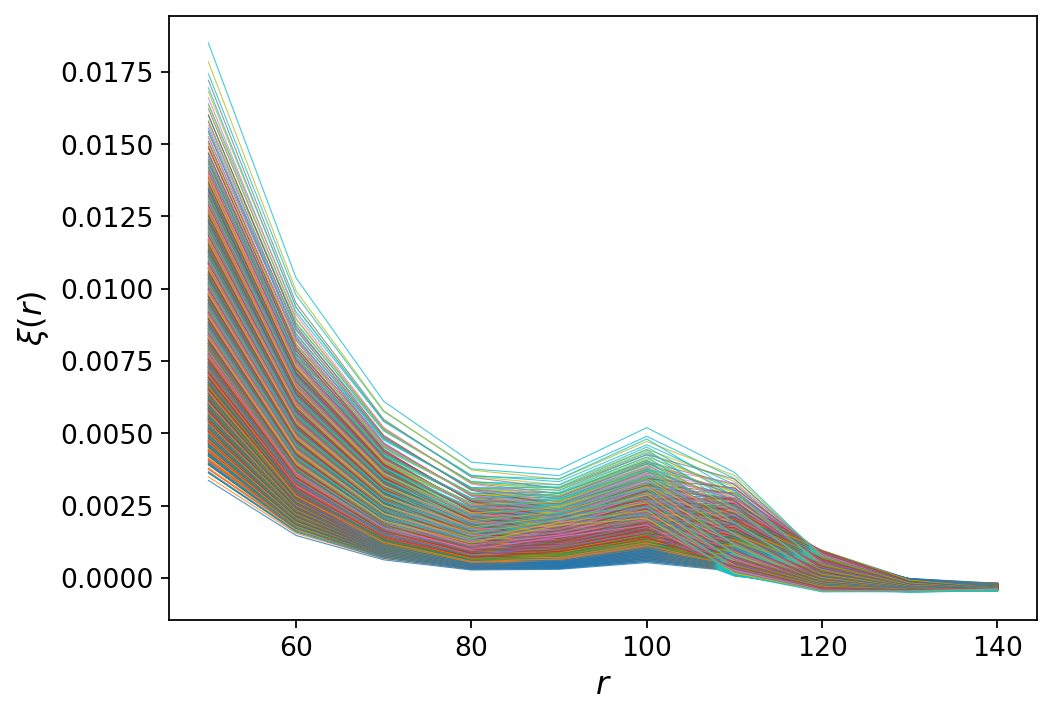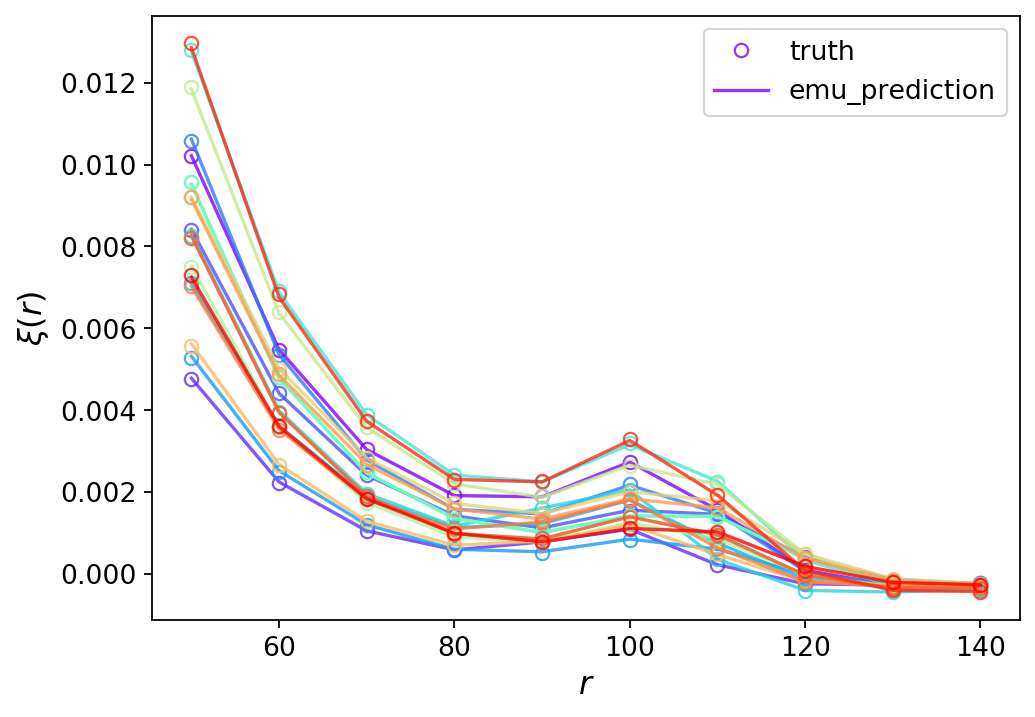# The Great Emu Fight¶

This notebook is the culmination of the emu-fight project, initiated at AstroHackWeek2020, by Kate Storey-Fisher, Catarina Alves, Johannes Heyl, Yssa Camacho-Neves, and Johnny Esteves.

## Preamble¶

Welcome one, welcome all, to the biggest fight of the gigayear: The Great Emu Fight!

No, we don't mean this emu fight:

In :
from IPython.display import Image
Image('images/emu_war.png', width=400)

Out:Nor do we mean this one:

In :
from IPython.display import HTML
HTML('<iframe width="560" height="315" src="https://www.youtube.com/embed/d9OBqYbZ99c" frameborder="0" allow="accelerometer; autoplay; encrypted-media; gyroscope; picture-in-picture" allowfullscreen></iframe>')

/Users/ksf/miniconda3/lib/python3.7/site-packages/IPython/core/display.py:717: UserWarning: Consider using IPython.display.IFrame instead

Out:

We're talking about emulators, or "emus" for short. Like the giant Australian birds, these emus are powerful and fast. Unlike the birds, our emus are regression models that are important for astrophysics, and they're a lot less fluffy.

In this tutorial, we'll teach you what an emulator is, and show you how to build emulators with various methods and frameworks. We will then pit our emus against each other and see which emu wins!

# 1. Theoretical Framework¶

## 1.1. What is an emulator?¶

Emulators imitate more complex models or simulations; they take input parameters and quickly spit out output values, without performing a physical calculation or a full simulation.

To do this, we train them on the inputs and outputs of simulations. They then learn this relationship, and for any new set of inputs, they can give an ouput. They are essentially fancy interpolators.

Let's take a simple example. Say a computational emu biologist wants to know how fast a particular emu can run. The biologist knows the emu's height, age, and fluffiness. They build a super intense simulator that takes in these demographics, and outputs the expected top speed of the bird.

The issue is that the biologist's simulator is so complicated that it takes an hour to run for each set of input parameters. This is where emulators come in. The biologist just runs a small number of simulations, sparsely spanning a reasonable range of parameter space (heights, ages, fluffiness levels). Then we train an emulator on this set of data. Now, for any other emu demographics, the emulator can quickly predict a given emu's top speed.

Why do we want an emulator of our simulation? They come in really handy for inference. Let's say we see an emu running at top speed down the street, breaking the city speed limit (yes, some can run this fast). But it got away, so to ID it we want to figure out its height, age, and fluffiness. If we only had the biologist's simulator, we would have to run a simulation at every possible demographic combination, and see which top speed most closely matched that of our runaway emu.

This would be untenable with the hour-long simulator. With our emulator, we can explore this parameter space much more quickly, and use techniques such as Markov Chain Monte Carlo to infer the distribution of likely bird characteristics.

## 1.2. Emulation in Astrophysics¶

Alas, we are not computational emu biologists but astrophysicists. But it turns out emulators are super handy for us too! Some areas emulators have been used:

• Emulating galaxy clustering statistics for cosmological parameter inference
• Emulating stellar population synthesis models
• Emulating supernovae spectra

Here we will demonstrate our emulators on the first use case. A common problem in cosmology is inferring the cosmological parameters that govern our universe - such as the overall density of matter and baryons. We can do this by running giant simulations of fake universes where we know the input cosmological parameters. We can then measure galaxy clustering statistics (e.g. a power spectrum) on both the real and fake universes, and compare them. However, we have the same problem here as we did with the emu speed simulator: it's very slow so we can't run a simulation and get statistics at all possible parameter sets.

We can instead run a small set of simulations spanning cosmological parameter space, and compute clustering statistics on these. Then we can train an emulator on these input parameters and output statistics, and use it to find a set of cosmological parameters that generates statistics most like those we measure in our real universe.

## 1.3. Our Dataset: The 2-Point Correlation Function¶

We generated a set of clustering statistics to demonstrate our emulators on. Instead of running full-blown cosmological simulations, which would take much longer than the span of this Hack Week, we used the nbodykit package. This can compute a model of a clustering statistic for a given cosmology in about a second - which is actually quite slow for robust inference, so our emulator is still useful!

We choose to emulate the 2-point correlation function, the Fourier transform of the power spectrum, which measures the strength of galaxy clustering at a given spatial scale.

We vary the cosmological parameters $\Omega_m$ (the matter density), $\sigma_8$ (the amplitude of clustering), and $\Omega_b$ (the baryon density), fixing the other parameters to reasonable values. We generate a training set of 8000 correlation functions, on a uniform 3D grid of these parameters (20 points per side). We also generate a test set of 100 correlation functions, to see how our emulator did.

# 2. Emulator war preparation¶

## 2.1. Import packages¶

In :
try:
import tensorflow
except ModuleNotFoundError:
!pip install tensorflow

In :
import timeit
import numpy as np
import matplotlib.pyplot as plt

In :
import emulator
%config InlineBackend.figure_format = 'retina'


## 2.2. Plot train set¶

Firstly, we plot the training set.

In :
emu = emulator.emulator()
path_train = 'data/cosmology_train.pickle'
path_test = 'data/cosmology_test.pickle'

In :
emu.plot_training()The pattern is because these are in order along the grid, so parameter sets near each other have similar shapes and are plotted in that order with corresponding colors.

## 2.3. The Emulator Lineup¶

We pit a few common emulator methods against each other:

• Artifical Neural Network
• Decision Tree
• Random Forest
• Gaussian Process
• Support Vectorm Machine

All of these are implemented in the emulator.py class; check it out for details on the implementation and hyperparameters. This class also performs some basic preprocessing, including normalizing/scaling the inputs and outputs for improved training.

For standalone notebooks constructing each of these emulators, see the linked jupyter notebooks.

We compare each on accuracy and speed. It would also be interesting to compare scaleability with respect to input parameter dimensionality; we leave this as an exercise to the reader. ;)

In :
run_timeit = True
from collections import defaultdict
time_dict = defaultdict(dict)

In :
regressor_names = emu.regressors_implemented
x = np.arange(len(regressor_names))
colors = np.array([plt.cm.Dark2(xx) for xx in x])


### 2.3.1 Artificial Neural Network Emulator¶

An Artificial Neural Network (ANN) is a class of machine learning methods loosely modeled after the neural connections in biological systems. It is also known as a Multi-Layer Perceptron (MLP), with layers that give various weights to input features. It uses an activation function to convert inputs to outputs and learn more complex functions. ANNs can be used for both classification and regression.

ANNs are useful for emulation as they can model complicated function spaces, with high-dimensional features. Here we use the scikitlearn implementation of an MLP regressor.

For a stand-alone walkthrough of constructing this emulator, see emulator_ANN_sklearn.ipynb.

In :
if run_timeit:
t = %timeit -o emu.train("ANN")
time_dict["ANN"]["train_mean"] = t.average
time_dict["ANN"]["train_std"] = t.stdev
else:
emu.train("ANN")

1.96 s ± 25.7 ms per loop (mean ± std. dev. of 7 runs, 1 loop each)

In :
if run_timeit:
t = %timeit -o emu.predict_test_set("ANN")
time_dict["ANN"]["test_mean"] = t.average
time_dict["ANN"]["test_std"] = t.stdev
else:
emu.predict_test_set("ANN")

1.09 ms ± 56.3 µs per loop (mean ± std. dev. of 7 runs, 1000 loops each)

In :
emu.plot_predictions("ANN")### 2.3.2. Decision Tree Emulator¶

Decision trees are regression or classification models in the form of a tree structure. They are among the most popular machine learning algorithms given their intelligibility and simplicity.

For a stand-alone walkthrough of constructing this emulator, see emulator_dtree.ipynb.

In :
if run_timeit:
t = %timeit -o emu.train("DTree")
time_dict["DTree"]["train_mean"] = t.average
time_dict["DTree"]["train_std"] = t.stdev
else:
emu.train("DTree")

138 ms ± 7.24 ms per loop (mean ± std. dev. of 7 runs, 10 loops each)

In :
if run_timeit:
t = %timeit -o emu.predict_test_set("DTree")
time_dict["DTree"]["test_mean"] = t.average
time_dict["DTree"]["test_std"] = t.stdev
else:
emu.predict_test_set("DTree")

700 µs ± 53.2 µs per loop (mean ± std. dev. of 7 runs, 1000 loops each)

In :
emu.plot_predictions("DTree")